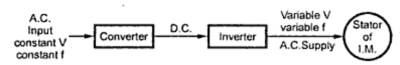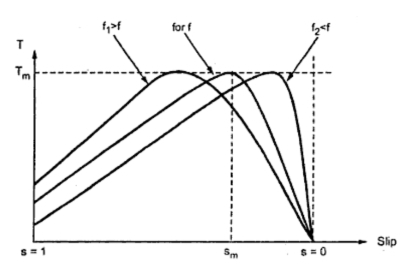### Supply Frequency Control or V / f Control

The synchronous speed is given by,
Ns = 120f / P
Thus by controlling the supply frequency smoothly, the synchronous speed can be controlled over a wide range. This gives smooth speed control of an induction motor.
But the expression for the air gap flux is given by,
This is according to the e.m.f. equation of a transformer where,
K1 = Stator winding constant
Tph1 = Stator turns per phase
V = Supply voltage
f = Supply frequency
It can be seen from this expression that if the supply frequency f is changed, the value of air gap flux also gets affected. This may result into saturation of stator and rotor cores. Such a saturation leads to the sharp increase in the (magnetisation) no load current of the motor. Hence it is necessary to maintain air gap flux constant when supply frequency f is changed.
To achieve this, it can be seen from the above expression that along with f, V also must be changed so as to keep (V/f) ratio constant. This ensures constant air gap flux giving speed control without affecting the performance of the motor. Hence this method is called V / f control.Fig. 1 Electronic scheme for V/f control
Hence in this method, the supply to the induction motor required is variable voltage variable frequency supply and can be achieved by an electronic scheme using converter and inverter circuitry. The scheme is shown in the Fig. 1.
The normal supply available is constant voltage constant frequency a.c. supply. The converter converts this supply into a d.c. supply. This d.c. supply is then given to the inverter. The inverter is a device which converts d.c. supply, to variable voltage variable frequency a.c. supply which is required to keep V / f ratio constant. By selecting the proper frequency and maintaining V / f constant, smooth speed control of the induction motor is possible.
If f is the normal working frequency then the Fig. 2 shows the torque-slip characteristics for the frequency f1 > f and f2 < f i.e. for frequencies above and below the normal frequency.Fig. 2 Torque-slip characteristics with variable f and constant (V/f)
Another disadvantages of this method is that the supply obtained can not be used to supply other devices which require constant voltage. Hence an individual scheme for a separate motor is required which makes it costly.